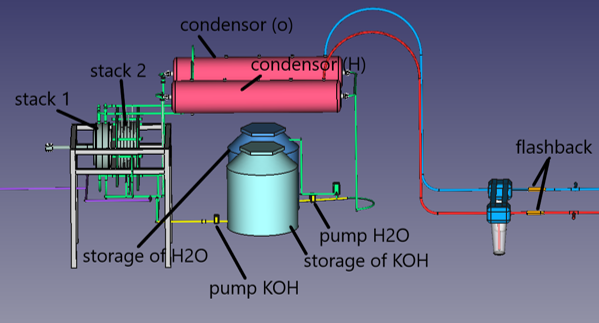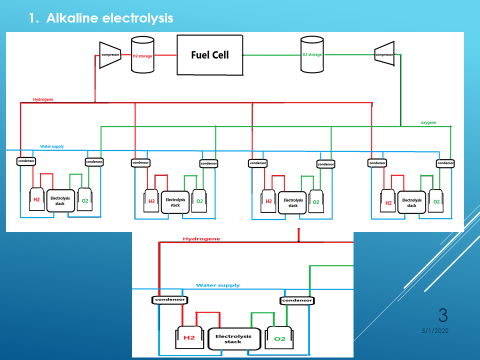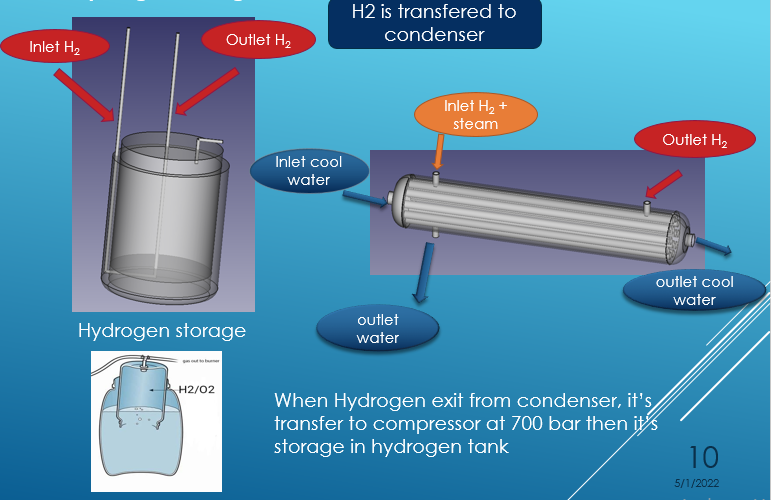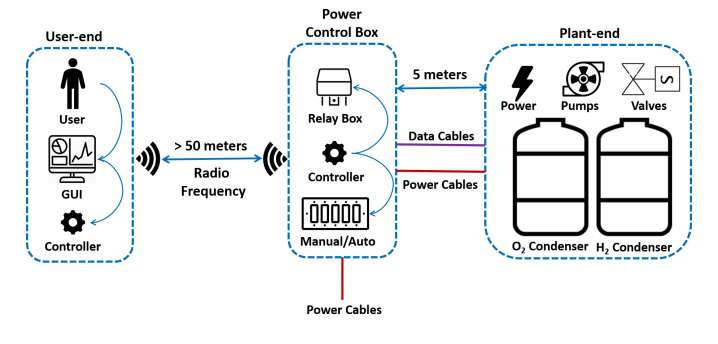# Electrolysis System Concept/ System Design

System Designcheck it here:

060419_1430_poster_multistack_electrolysis.pdf

Mechanical DesignMechanical DesignHydrogen Storage:check the full presentation: 050419_ElectrolysisOfWater.pptx

PCS DesignPCS Design

Needed inormations for the design and the calculations :

The proposed spaces were 10.65, 9.20, 8.25, 7.25, 6.30, 6.05, 4.35, 4.15, and 3.40 millimetres. From the nine different analysed distances between electrodes, it can be said that the best performance was reached by one of the smallest distances proposed, 4.15 mm. When the same distance between electrodes was compared (the same and different distance between electrodes and separator), the one that had almost twice the distance (negative compartment) presented an increase in current density of approximately 33% with respect to that where both distances (from electrodes to separator) are the same. That indicates that the stichometry of the electrolysis reaction influenced the performance.L = A x R/ρ.

R = L x ρ / A = L / (A x K )

K =1/ρ  .

U= R×I

current density i nalkaline electrolysis= 0,2-0,4 A/cm square

# calculating the current and the voltage for the existing cells

the bigger cell in which the distance between the eectrodes is 3,6 cm

cell details :

The radius of the surface which touches the solution : 14,6 cm .

The distance between the electrode and the membrane : 3,6 cm .

We filled only 2/3 of the cell volume .

Temperature = 25 degree celsius

So R =  (3,6 cm)/((446,21 cm square × 0,125 ))  = 0,0645 Ω .

I = 178,484 Amperes

U = 11,52 Volt

the smaller cells in which the distance between the eectrodes is 1,8 cm

L = 1,8 cm

So  R =(1,8 cm)/((446,21 cm square × 0,125 ))  = 0,0322 Ω

I = 178,484 Amperes

U =5,747 volt

# calculating the current the voltage and the distance between electrodes of the multistage electrolyser cell :

L= A x  ( 6)/(A )  x 0,3375 = 2,025 cm

I = 0,4 x the surface that touches the solution=0,4 x A=0,4×3,14×r×r

U = 2,4 Volt

So the distance betwee the two electrodes shall be 2,025 cm .

And from the text above we seen that it is better to divide this distance into 3 parts

2 parts on the hydrogen production site and one on the oxygene production .

That means 0,675 cm from the anode to the membrane and 1,35 cm from the caathode to the membrane .

References :

Related Sites/Items:

 System Specification Mechanical Design Electrolyser Mechanical Design Process Control System Spec./Design PCS Design Mechanical Realization Electrolyser Mechanical Realization Process Control System Realization (PLC+GUI) PCS Realization System Test Specification Test Specification System Testing Test Cases